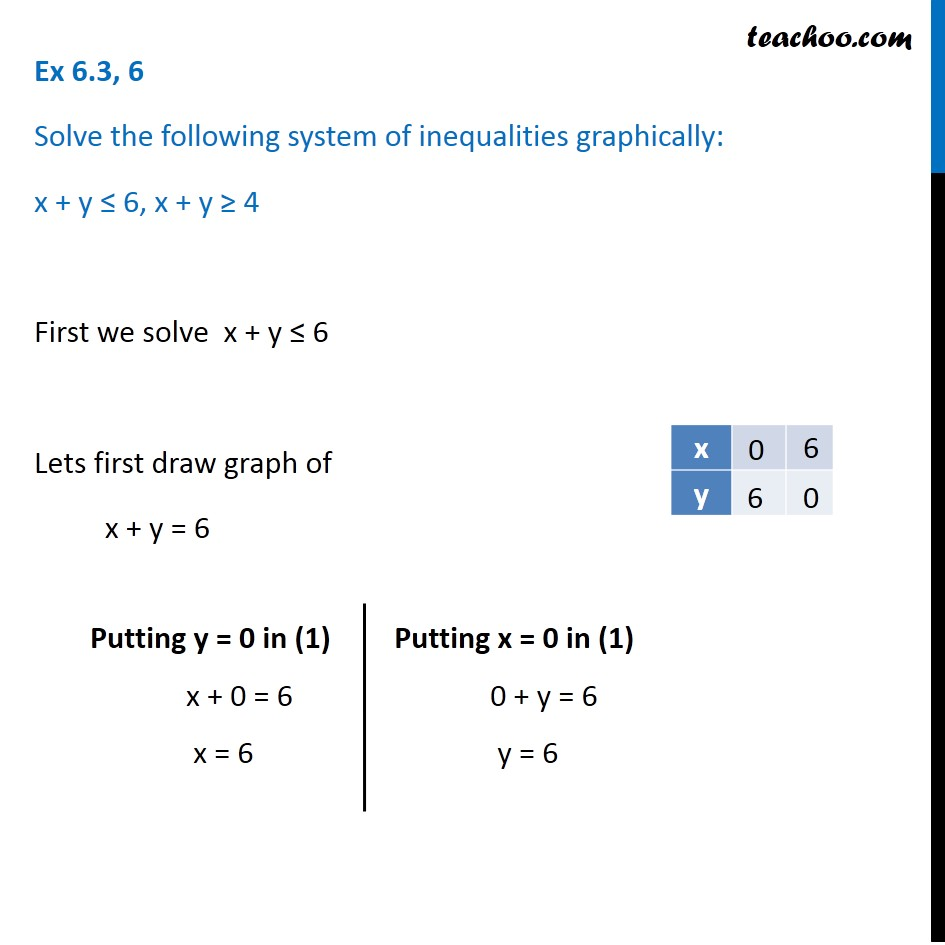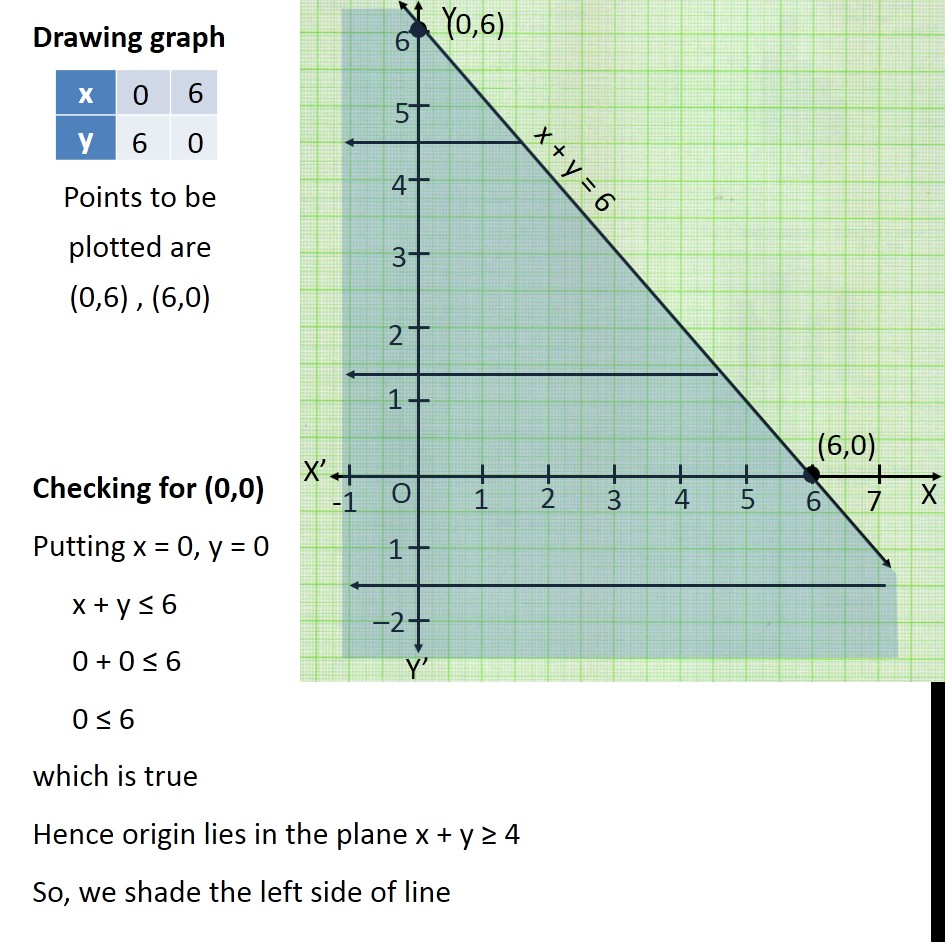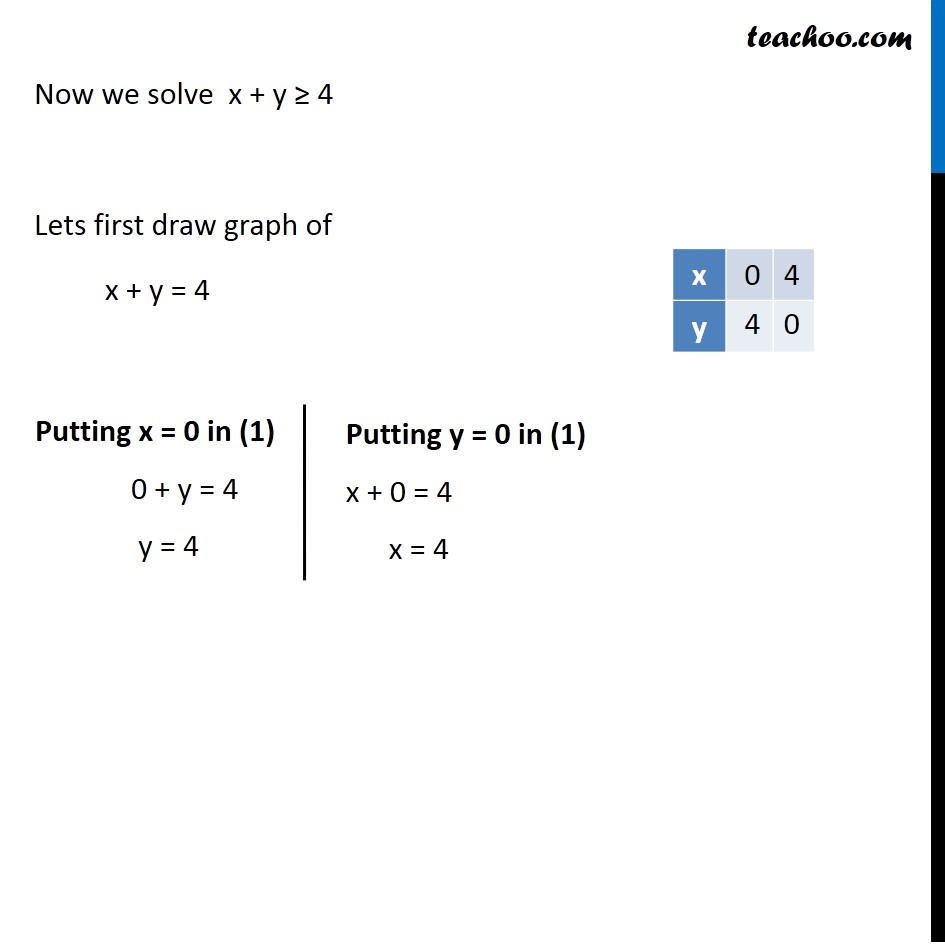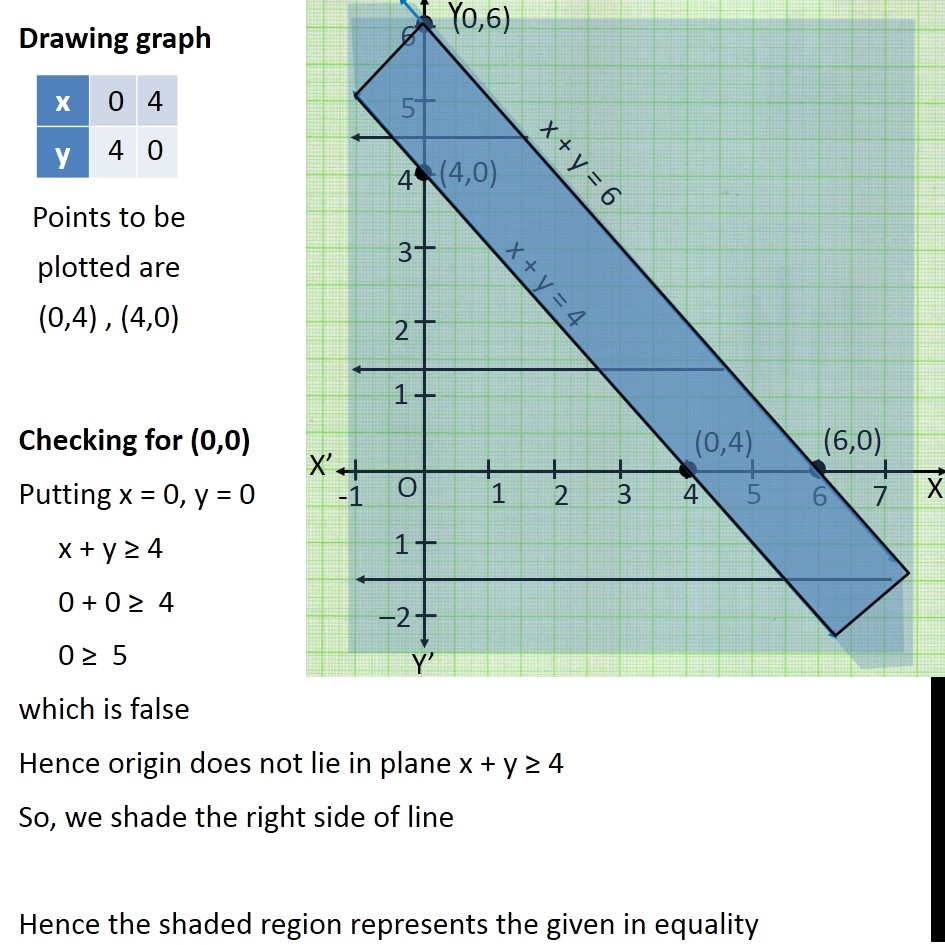Solving Pair of Linear Inequalities

Chapter 5 Class 11 Linear Inequalities
Serial order wiseLearn in your speed, with individual attention - Teachoo Maths 1-on-1 Class

### Transcript

Question 6 Solve the following system of inequalities graphically: x + y ≤ 6, x + y ≥ 4 First we solve x + y ≤ 6 Lets first draw graph of x + y = 6 Putting y = 0 in (1) x + 0 = 6 x = 6 Putting x = 0 in (1) 0 + y = 6 y = 6 Points to be plotted are (0,6) , (6,0) Drawing graph Checking for (0,0) Putting x = 0, y = 0 x + y ≤ 6 0 + 0 ≤ 6 0 ≤ 6 which is true Hence origin lies in the plane x + y ≥ 4 So, we shade the left side of line Now we solve x + y ≥ 4 Lets first draw graph of x + y = 4 Putting x = 0 in (1) 0 + y = 4 y = 4 Putting y = 0 in (1) x + 0 = 4 x = 4 Drawing graph Checking for (0,0) Putting x = 0, y = 0 x + y ≥ 4 0 + 0 ≥ 4 0 ≥ 5 which is false Hence origin does not lie in plane x + y ≥ 4 So, we shade the right side of line Hence the shaded region represents the given in equality Points to be plotted are (0,4) , (4,0)